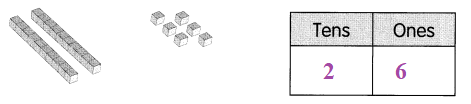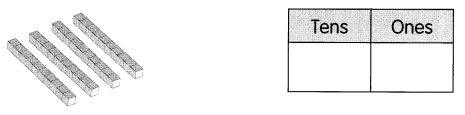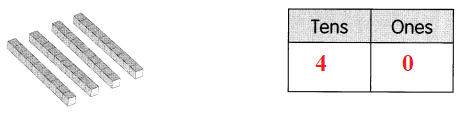# Math in Focus Grade 1 Chapter 12 Practice 2 Answer Key Place Value

Practice the problems of Math in Focus Grade 1 Workbook Answer Key Chapter 12 Practice 2 Place Value to score better marks in the exam.

## Math in Focus Grade 1 Chapter 12 Practice 2 Answer Key Place Value

Fill in the missing numbers.

Question 1.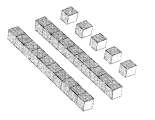25 = ___ tens ___ ones
Answer: 25 = 2 tens 5 ones

Question 2.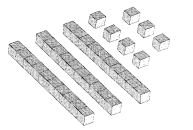37 = ___ tens __ ones
Answer: 37 = 3 tens 7 ones

Look at each place-value chart. Write the number it shows.

Question 3.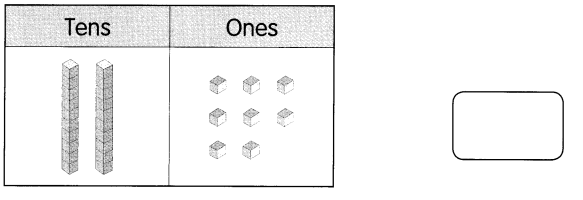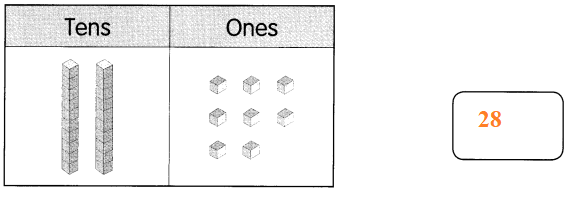Question 4.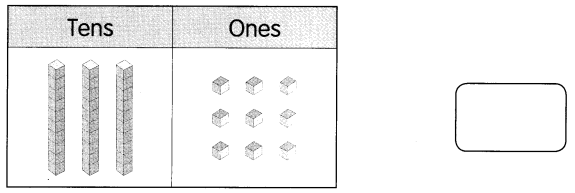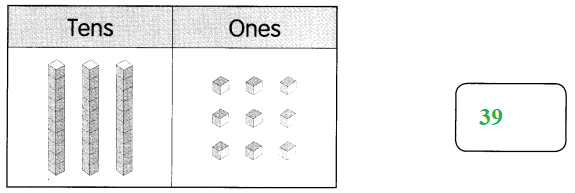Count in tens and ones. Fill in the missing numbers ¡n the place-value charts and blanks.

Example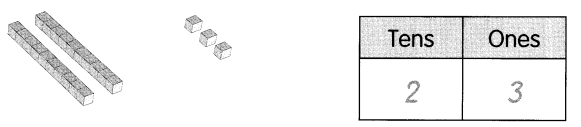23 = 2 tens 3 ones
20 + 3 = 23

Question 5.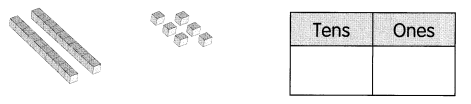26 = ____ tens ___ ones
20 + 6 = ____